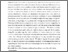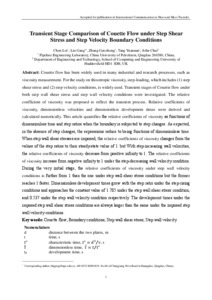Chen, Lei, Liu, Gang, Zhang, Guozhong, Tang, Yuannan and Chai, John (2016) Transient Stage Comparison of Couette Flow under Step Shear Stress and Step Velocity Boundary Conditions. International Communications in Heat and Mass Transfer, 75. pp. 232-239. ISSN 0735-1933
Abstract

Couette flow has been widely used in many industrial and research processes, such as viscosity measurement. For the study on thixotropic viscosity, step-loading, which includes (1) step shear stress and (2) step velocity conditions, is widely used. Transient stages of Couette flow under both step wall shear stress and step wall velocity conditions were investigated. The relative coefficient of viscosity was proposed to reflect the transient process. Relative coefficients of viscosity, dimensionless velocities and dimensionless development times were derived and calculated numerically. This article quantifies the relative coefficients of viscosity as functions of dimensionless time and step ratios when the boundary is subjected to step changes. As expected, in the absence of step changes, the expressions reduce to being functions of dimensionless time. When step wall shear stresses are imposed, the relative coefficients of viscosity changes from the values of the step ratios to their steady-state value of 1. but With step-increasing wall velocities, the relative coefficients of viscosity decrease from positive infinity to 1. The relative coefficients of viscosity increase from negative infinity to 1 under the step-decreasing wall velocity condition. During the very initial stage, the relative coefficients of viscosity under step wall velocity conditions is further from 1 than the one under step wall shear stress conditions but the former reaches 1 faster. Dimensionless development times grow with the step ratio under the step-rising conditions and approaches the constant value of 1.785 under the step wall shear stress condition, and 0.537 under the step wall velocity condition respectively. The development times under the imposed step wall shear stress conditions are always larger than the same under the imposed step wall velocity conditions.

Information
Library
DocumentsPreview
ICHMT.pdf - Accepted Version